# RD Sharma Solutions - Chapter 2 - Powers (Ex-2.2) Part - 1, Class 8 Math Notes | Study RD Sharma Solutions for Class 8 Mathematics - Class 8

## Class 8: RD Sharma Solutions - Chapter 2 - Powers (Ex-2.2) Part - 1, Class 8 Math Notes | Study RD Sharma Solutions for Class 8 Mathematics - Class 8

The document RD Sharma Solutions - Chapter 2 - Powers (Ex-2.2) Part - 1, Class 8 Math Notes | Study RD Sharma Solutions for Class 8 Mathematics - Class 8 is a part of the Class 8 Course RD Sharma Solutions for Class 8 Mathematics.
All you need of Class 8 at this link: Class 8

Question 1:Write each of the following in exponential form: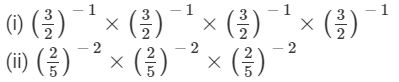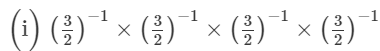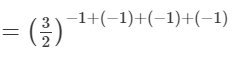{am×an=am+n}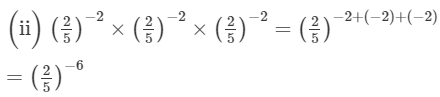{am×an=am+n}
Question 2:
Evaluate:
(i) 5−2
(ii) (−3)−2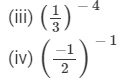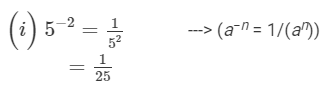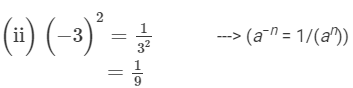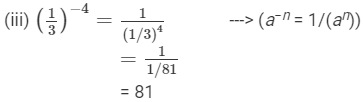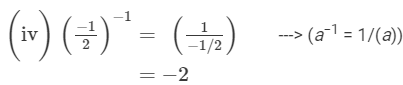Question 3: Express each of the following as a rational number in the form p/q:

(i) 6−1
(ii) (−7)−1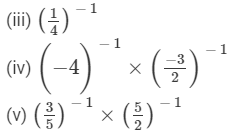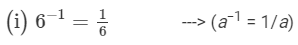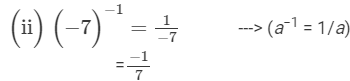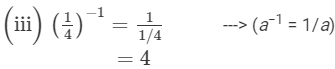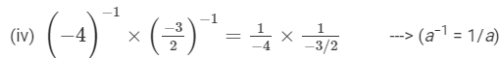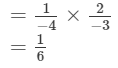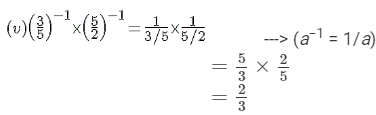Question 4:
Simplify:

(i) {4−1×3−1}2

(ii) {5−1÷6−1}3
(iii) (2−1+3−1)−1
(iv) {3−1×4−1}−1×5−1
(v) (4−1−5−1)÷3−1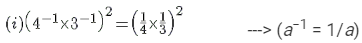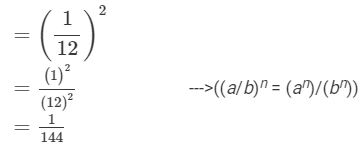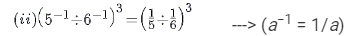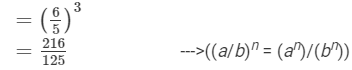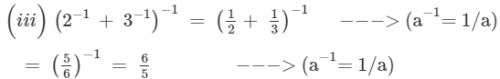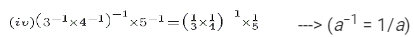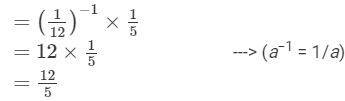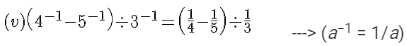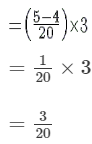Question 5: Express each of the following rational numbers with a negative exponent: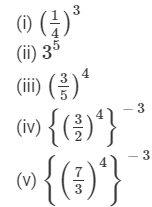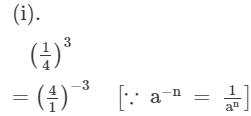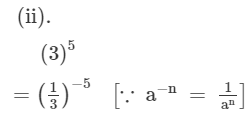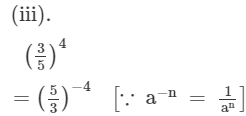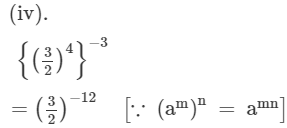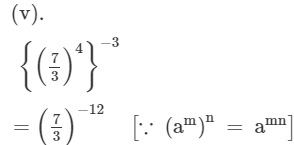Question 6: Express each of the following rational numbers with a positive exponent: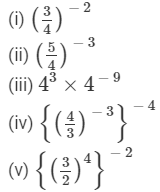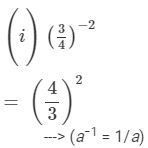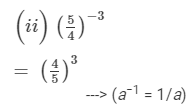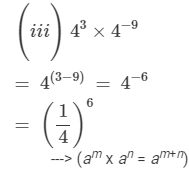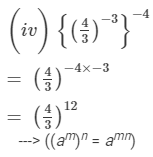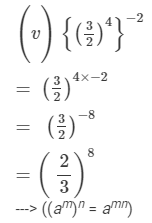Question 7:

Simplify: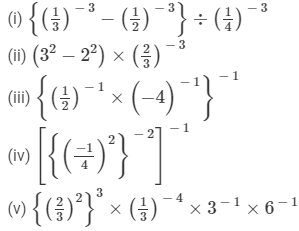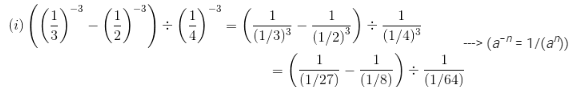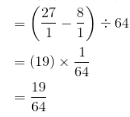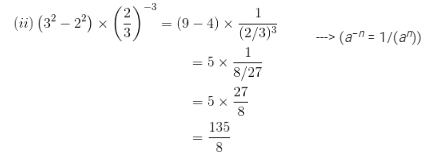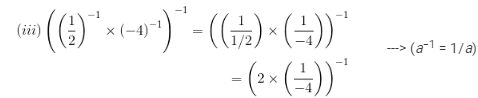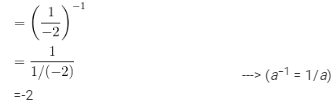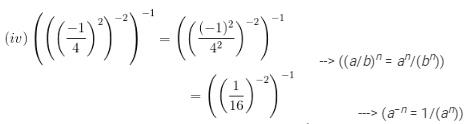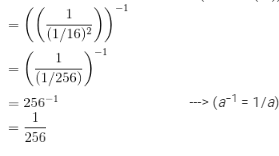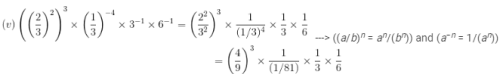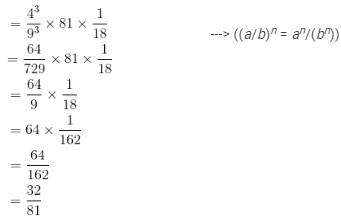The document RD Sharma Solutions - Chapter 2 - Powers (Ex-2.2) Part - 1, Class 8 Math Notes | Study RD Sharma Solutions for Class 8 Mathematics - Class 8 is a part of the Class 8 Course RD Sharma Solutions for Class 8 Mathematics.
All you need of Class 8 at this link: Class 8Use Code STAYHOME200 and get INR 200 additional OFF

## RD Sharma Solutions for Class 8 Mathematics

88 docs

Track your progress, build streaks, highlight & save important lessons and more!

,

,

,

,

,

,

,

,

,

,

,

,

,

,

,

,

,

,

,

,

,

,

,

,

;